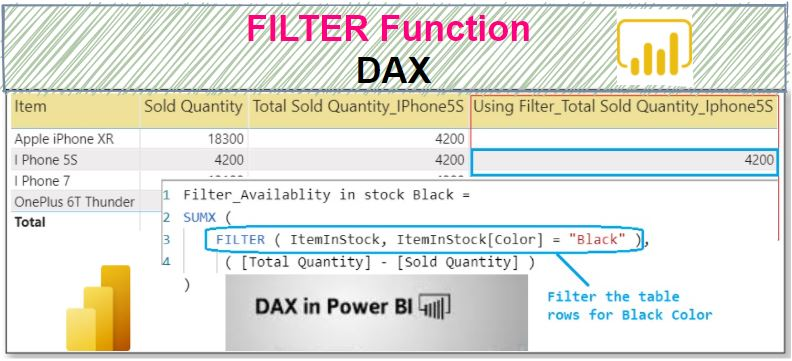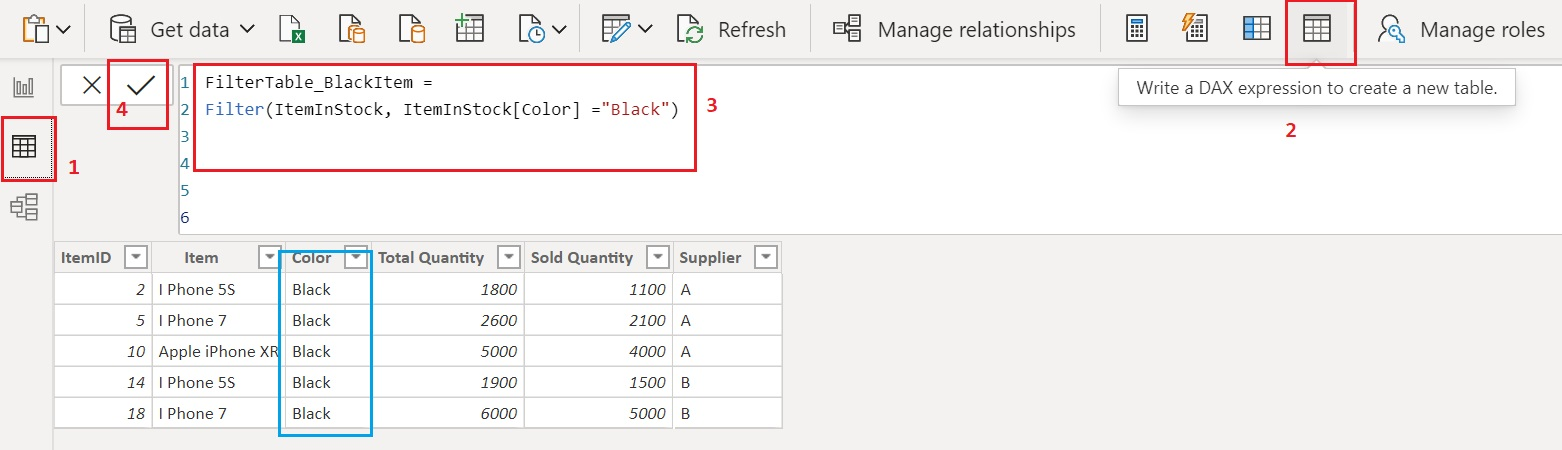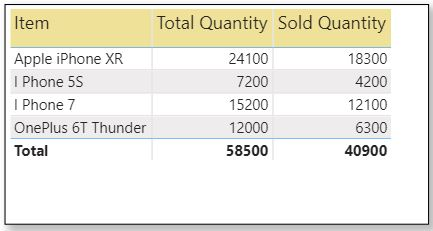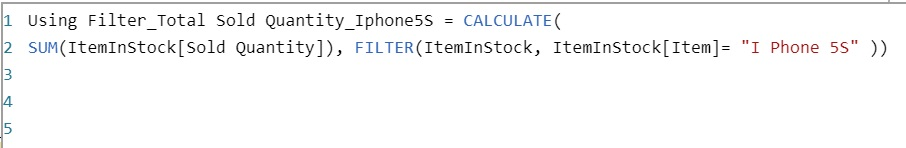Home » FILTER Function DAX

# FILTER Function DAX### Filter is a Power BI filter functions in DAX which returns a table that represents a subset of another table or expression.

It filter the table under current filter context.

It returns the table that has the same columns as in the original table but contains only those rows which satisfied filter condition applied row by row.

DAX SYNTAX

`FILTER(<table>,<filter>)`

table is the table to be filtered. The table can also be an expression that results in a table.
filter is a Boolean expression that is to be evaluated for each row of the table.

Lets look at an example of using Filter in Power BI.

Here we have a sample table named Item, which contains the details of sold items by supplier.#### Creating a new table using FITLER function

Lets use the filter function that will filter the item table for black color phone and returns a new table.

In Data view, click on Create a new table icon then write a following DAX.

```FilterTable_BlackItem =

Filter(ItemInStock, ItemInStock[Color] ="Black")```Once you commit the measure, you can see it returns a new table named FilterTable_BlackItem that contains rows only for back color phone.

#### Using a FILTER function in measure

Following are the summary report of sold items, which display the Items by total quantity and Sold quantity as shown below.Suppose you want to see an additional details in the above summary report that is the total available quantity in stock for each phone model also how much quantity of black color phone has left in stock for those model.

For this requirement you need to go through two major steps as follows:

Step 1: You need to create a measure to calculate to get the Total Available quantity in stock, which will be calculated as Total Quantity – Total Sold Quantity.

Lets create a measure named Total Availability in stock as following.

```Total Availability in Stock =

SUMX(ItemInStock, ItemInStock[Total Quantity] - ItemInStock[Sold Quantity])```

Now commit the measure and drag into table visual next to the Sold Quantity field to see the output.

As you can see it returns the Total Available quantity for each model.Step 2: Now to see the Total Available quantity in stock for black color in each model, you need create another measure that will calculate the total available quantity only of black color phone for each model as following.

```Filter_Availablity in stock Black =
SUMX (
FILTER ( ItemInStock, ItemInStock[Color] = "Black" ),
( [Total Availability in Stock])
)```Lets commit the measure and drag into table visual to see the output.

As you can see, it returns the availability of black color for each model. Also you can see there is no black color available for phone model Oneplus 6T Thunder.Lets understand the behaviour of Measure Filter_Availablity in stock Black.

SUMX function requires first argument as table, for that you have provided a table using filter functions which returns the table by filtering a rows in table for black color.

For second argument, it requires an expression for that you have provided a measure Total Availability in Stock which is evaluated for each row in a table and returns the total available quantity for black in each model.

Now you can easily see the Total Quantity left in stock as well as the quantity of black color for each model together.

#### Using FILTER function with CALCULATE function

Lets use simple calculate function which returns the total sold quantity for Item I phone 5S.

Following measure named Total Sold Quantity_IPhone5S uses CALCULATE function and returns the total Sold Quantity of black color model in each model.

```Total Sold Quantity_IPhone5S =
CALCULATE ( SUM ( ItemInStock[Sold Quantity] ), ItemInStock[Item] = "I Phone 5S" )```Once you commit the measure, Lets take this measure into table visual to see the Total Sold Quantity for I phone 5S.As you can see, using CALCULATE function, the Item is filtered for I phone 5S that means the filter is applied to all the rows and there is no filter to the table so it returns the total sold quantity of I Phone 5S for other Items as well.
Lets use FILTER function with CALCULATE function.
```Using Filter_Total Sold Quantity_Iphone5S = CALCULATE(

SUM(ItemInStock[Sold Quantity]), FILTER(ItemInStock, ItemInStock[Item]= "I Phone 5S" ))```As you can see, using a FILTER function which returns a table, filters the rows for Item I Phone 5S in table then SUM function is applied on that result table and returns the Total Sold Quantity for item I phone 5S only.